﻿ SQL IN Clause - TutorialsTrend
This Angular tutorial helps you get started with Angular quickly and effectively through many practical examples.
`

# SQL-IN Clause

The IN operator is a logical operator that allows you to test whether a specified value matches any value in a list. It is used to remove the need for multiple OR conditions in SELECT, INSERT, UPDATE, or DELETE. The IN operator allows you to specify multiple values in a WHERE clause. In this tutorial we will see how to use IN operator in place of multiple OR operator.

Syntax

``````SELECT column_name1, column_name2 FROM table_name
WHERE column_name || expression IN (value1,value 2)

or

SELECT column_name1, column_name2
FROM table_name
WHERE column_name IN (SELECT STATEMENT ); ``````

If a value in the column or the expression is equal to any value in the list, the result of the IN operator is TRUE.

For the demo purpose, we will use the below table. The table looks like that: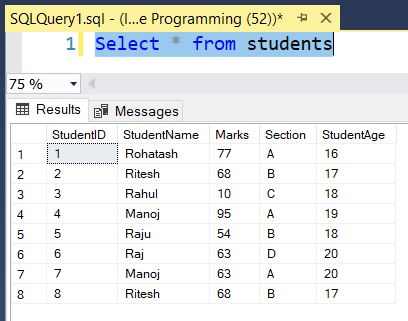#### SQL Server IN with List Values

The following statement finds the students whose name are as following: 'manoj', 'Rohatash', 'Raj'. the following query that uses the OR operator.

``````SELECT StudentName, Marks, StudentAge
FROM students
WHERE StudentName = 'manoj' OR StudentName = 'Rohatash' OR StudentName ='Raj'
ORDER BY StudentID;``````

The result is as follows: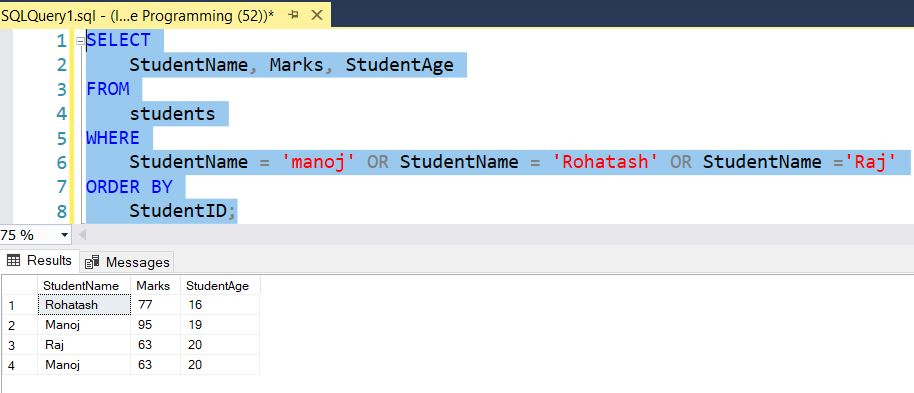The query above is equivalent to the following query that uses the IN operator instead:

``````SELECT StudentName, Marks, StudentAge
FROM students
WHERE StudentName in('manoj', 'Rohatash', 'Raj')
ORDER BY StudentID;``````

The result is as follows: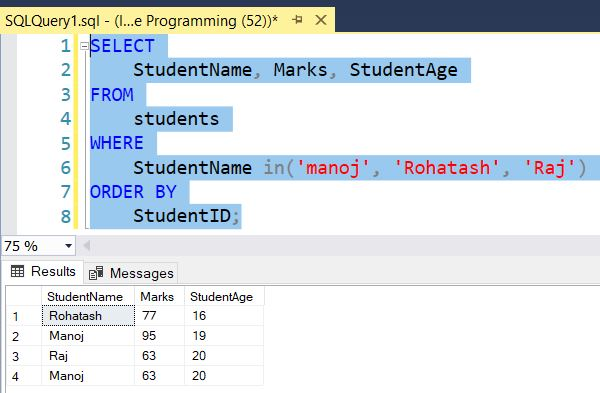#### NOT IN Operator

The NOT IN operator returns is used to filter the rows that are not match values in the list. It returns all the rows except the excluded rows.

For example,

``````SELECT StudentName, Marks, StudentAge
FROM students
WHERE StudentName not in('manoj', 'Rohatash', 'Raj')
ORDER BY StudentID;``````

The result is as follows: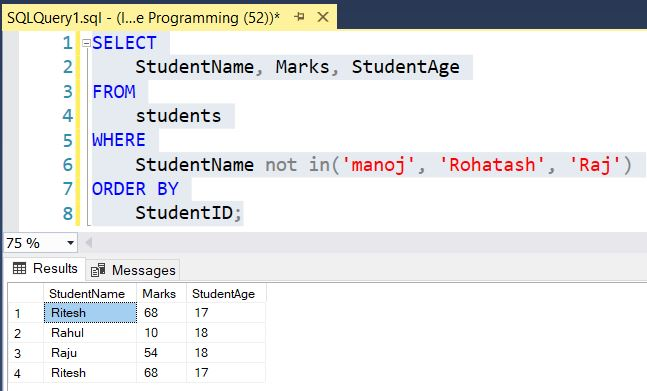#### SQL Server IN Operator with Subquery

You can use the subquery with the IN operator that returns records from the single column. The subquery cannot include more than one column in the SELECT column list.

Here, select rows from students table where StudentID is in the result set of subquery.

For Example

``````SELECT StudentName, Marks, StudentAge
FROM students
WHERE StudentID in (
SELECT StudentID FROM students WHERE StudentName = 'manoj' or StudentName = 'Rohatash' or StudentName='Raj'
)
ORDER BY StudentID;``````

The result is as follows: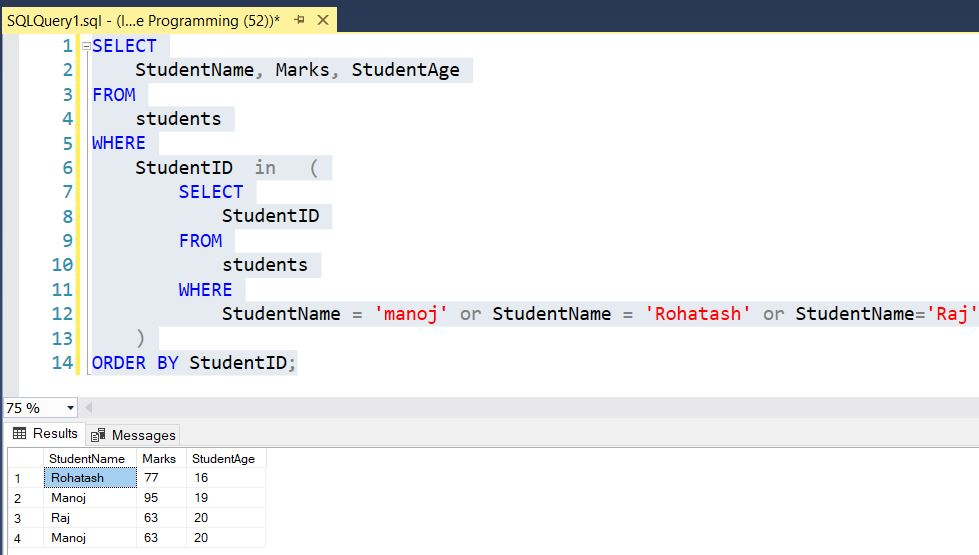Next